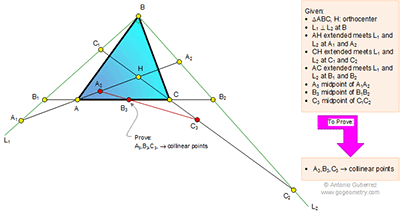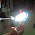## Tuesday, June 16, 2015

### Geometry Problem 1122: Triangle, 90 Degree, Perpendicular Lines, Orthocenter, Altitude, Midpoint, Collinear Points

Level: Mathematics Education, High School, Honors Geometry, College.

Click the diagram below to enlarge it.1.2.This is quite clearly a Cartesian coordinate question in disguise. The diagram has been rotated and the “orthocenter” has been introduced, but it’s under the difficulty of a Year 10 problem.
Let A1B be the x axis and BC2 be the y axis. B = (0, 0).
H is the orthocenter, which means that AH is perpendicular to BC and AB is perpendicular to CH.
Let A = (p, q) and C = (r, t).
Using the two – point form and point-gradient form of a straight line, we find that:
The line AC takes the form: x(t – q) – y(r – p) + (rq – pt) = 0
The line AH takes the form (-r/t)(x – p) = y – q
The line CH takes the form (-p/q)(x – r) = y – t
We find A2, B2, etc by finding the x and y intercepts of each of the above equations.
A1 = ((tq + pr)/r, 0), A2 = (0, (qt+rp)/t), B1 = ((pt-rq)/(t-q), 0) B2 = (0, (rq-pt)/(r-p))
C1 = ((qt+rp)/p, 0) C2 = (0, (pr+qt)/q)

Then we find the relevant midpoints.
A3 = ((qt + pr)/2r, (qt + pr)/2t) B3 = (0.5 (rq-pt)/(q-t), 0.5(rq-pt)/(r-p))
C3 = ((qt + pr)/2p, (qt + pr)/2q)

Now all we need to do is to plug this into the shoelace formula. If A3, B3, C3 are collinear, then the determinant will evaluate to be zero (as the area formed by A3, B3, C3 will be zero).

The matrix determinant does indeed evaluate to zero (and readers are free to try it), hence the proof is complete.